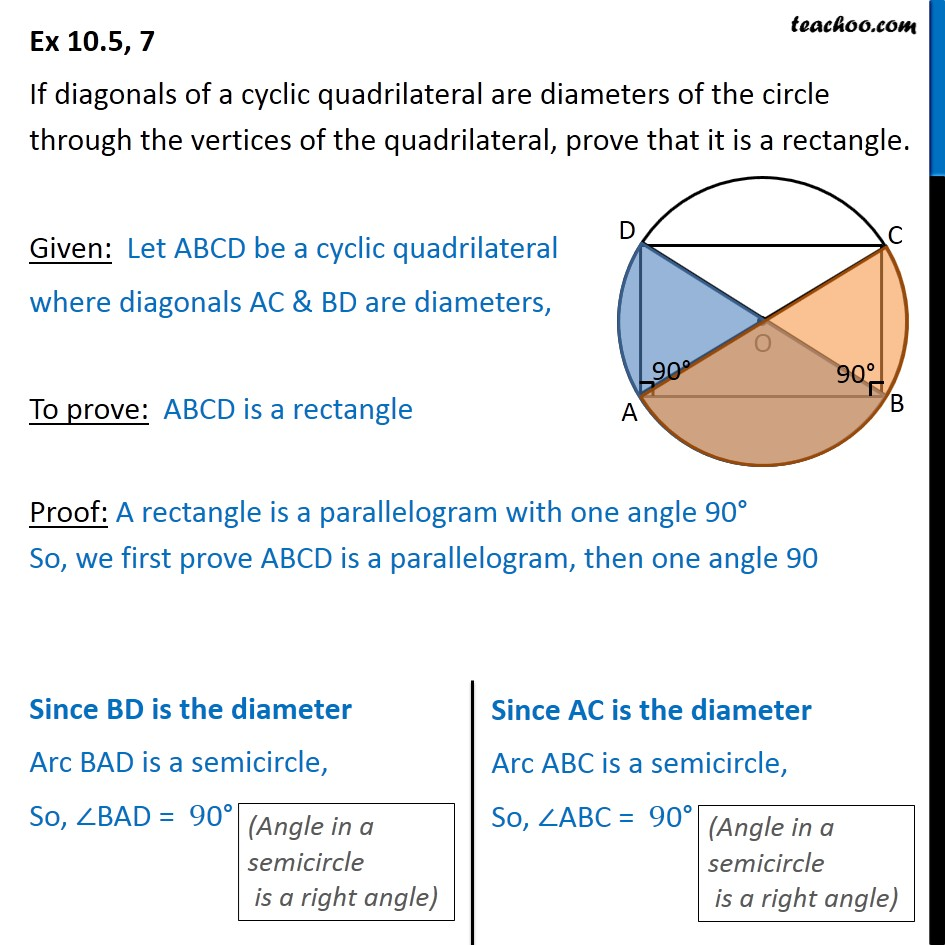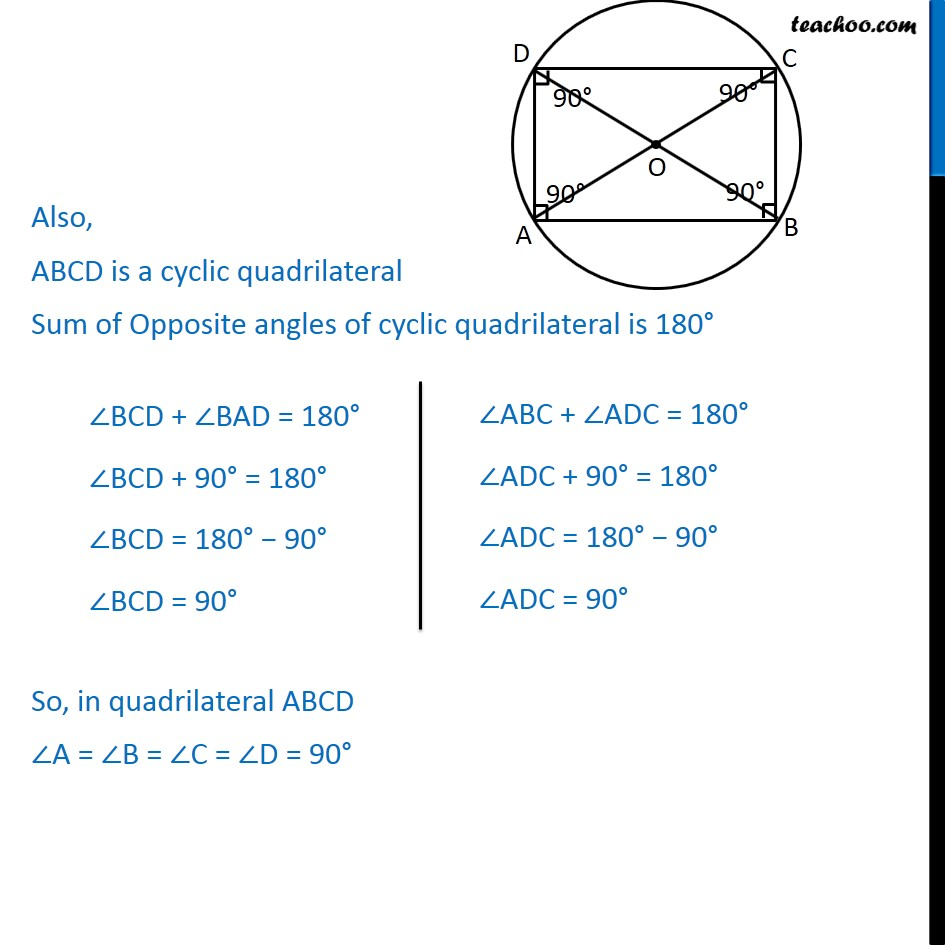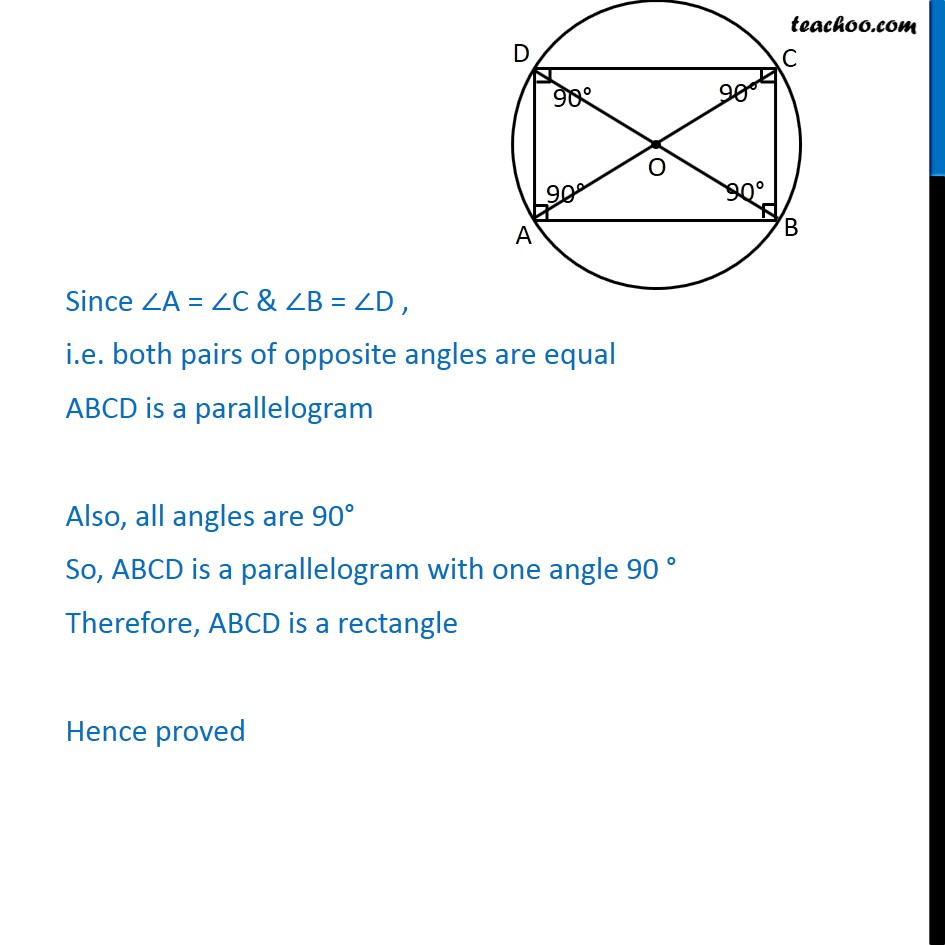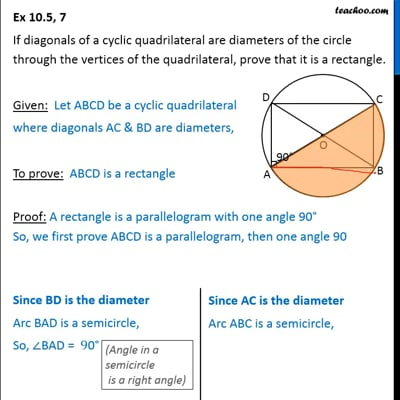Ex 9.3

Chapter 9 Class 9 Circles
Serial order wiseThis video is only available for Teachoo black users

Learn in your speed, with individual attention - Teachoo Maths 1-on-1 Class

### Transcript

Ex 9.3, 7 If diagonals of a cyclic quadrilateral are diameters of the circle through the vertices of the quadrilateral, prove that it is a rectangle. Given: Let ABCD be a cyclic quadrilateral where diagonals AC & BD are diameters, To prove: ABCD is a rectangle Proof: A rectangle is a parallelogram with one angle 90 So, we first prove ABCD is a parallelogram, then one angle 90 Since BD is the diameter Arc BAD is a semicircle, So, BAD = 90 Also, ABCD is a cyclic quadrilateral Sum of Opposite angles of cyclic quadrilateral is 180 So, in quadrilateral ABCD A = B = C = D = 90 Since A = C & B = D , i.e. both pairs of opposite angles are equal ABCD is a parallelogram Also, all angles are 90 So, ABCD is a parallelogram with one angle 90 Therefore, ABCD is a rectangle Hence proved